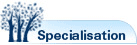# Mathematics

## Description

The discipline of mathematics at UNSW is divided into several areas of study: Pure Mathematics, Applied Mathematics and Statistics.

Pure Mathematics is the study of the essential structures of mathematics. Work by pure mathematicians underpins most of the technological advances of this century. Pure Mathematics is concerned with problems and techniques which transcend specific applications. Research, focusing on the development of existing theories or the creation of new ones, may be driven by applications or by the internal demands of the discipline. Pure Mathematics courses provide the insights and understanding required by those using mathematics, leading to mastery of the fundamental processes of mathematical science and the capacity for innovative applications in any area.

Applied Mathematics concerns the development of mathematics and models for understanding scientific phenomena, for the solution of technical and industrial problems, and for use in the social, economic and management sciences. Courses are designed to provide basic mathematical and computational skills needed for a wide range of applications, to develop the capability to construct, analyse and interpret mathematical models, and to encourage enthusiasm for the role of the mathematician in a variety of contexts.

Statistics is the science and art of using factual material for modelling and inference. Its mathematical foundations are in the theory of probability and it deals with how to estimate and make decisions using knowledge which is uncertain or observational material which is subject to error. There is a rich interplay of ideas between the theory of statistics and fields such as engineering, medicine and biological and behavioural sciences where statistical problems constantly arise.

Studying Mathematics at UNSW

The study of mathematics at UNSW is primarily through the School of Mathematics and Statistics. Please refer to the table below for a list of research programs available at UNSW.

#### Mathematics can be studied in the following Programs

Program Code & Name Stream Type Stream
1001 Cotutelle Research MATHMR1001
1880 Mathematics Research MATHMR1880
2920 Mathematics Research MATHMR2920